# Training and hyperparameter tuning a PyTorch model on Cloud AI Platform

## 1. Overview

In this lab, you will walk through a complete ML training workflow on Google Cloud, using PyTorch to build your model. From a Cloud AI Platform Notebooks environment, you'll learn how to package up your training job to run it on AI Platform Training with hyperparameter tuning.

## What you learn

You'll learn how to:

• Create an AI Platform Notebooks instance
• Create a PyTorch model
• Train your model with hyperparameter tuning on AI Platform Training

The total cost to run this lab on Google Cloud is about \$1.

## 2. Setup your environment

You'll need a Google Cloud Platform project with billing enabled to run this codelab. To create a project, follow the instructions here.

## Step 1: Enable the Cloud AI Platform Models API

Navigate to the AI Platform Models section of your Cloud Console and click Enable if it isn't already enabled.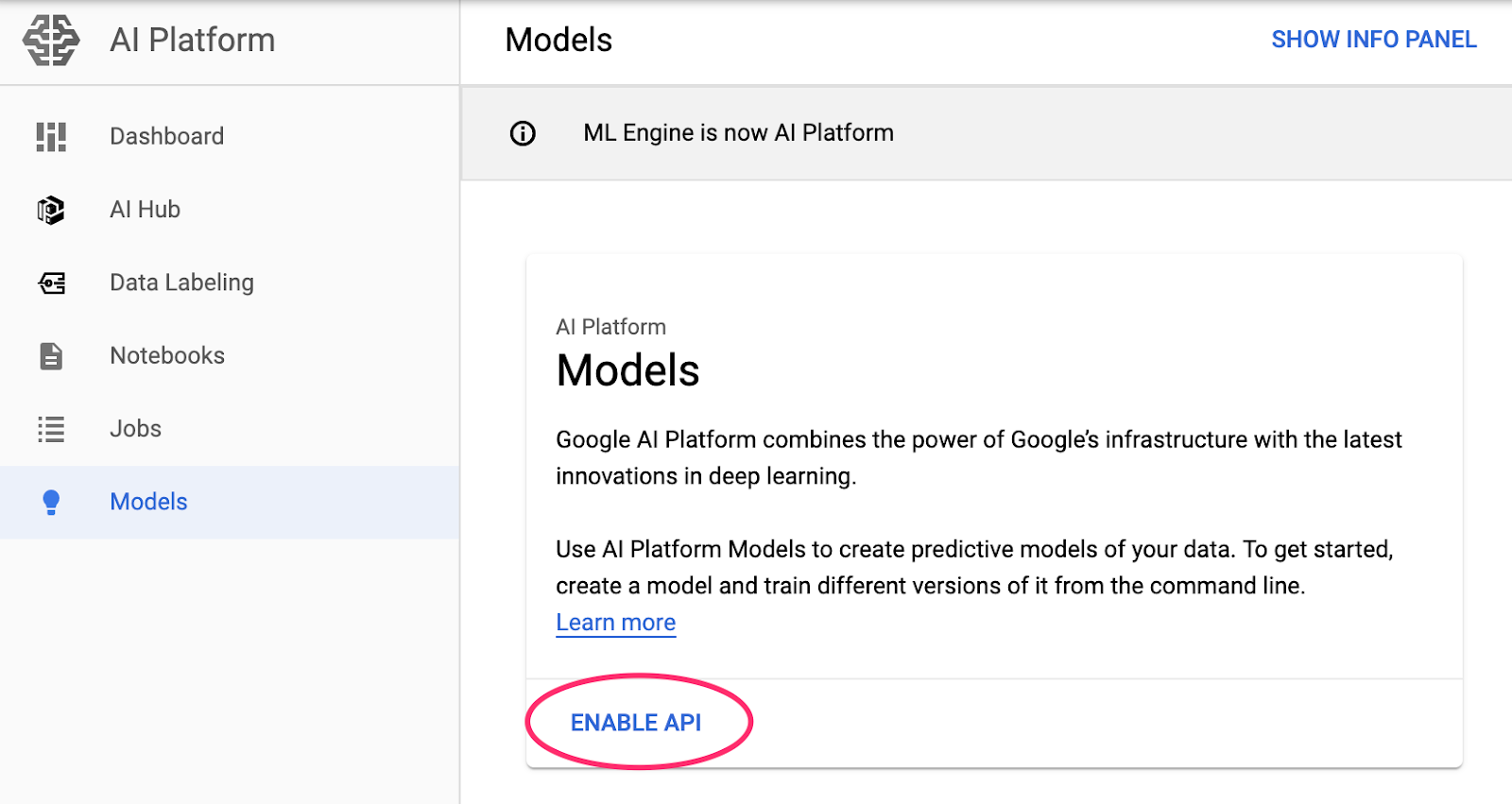## Step 2: Enable the Compute Engine API

Navigate to Compute Engine and select Enable if it isn't already enabled. You'll need this to create your notebook instance.

## Step 3: Create an AI Platform Notebooks instance

Navigate to AI Platform Notebooks section of your Cloud Console and click New Instance. Then select the latest PyTorch instance type (without GPUs):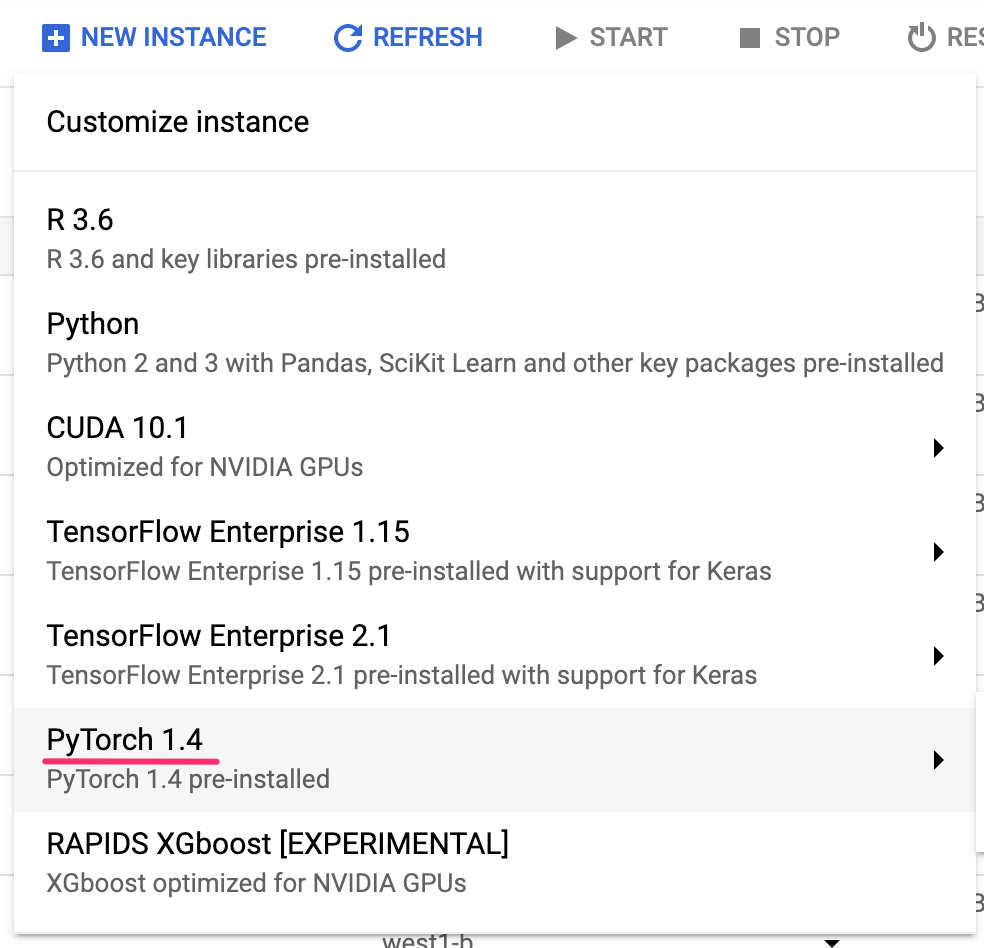Use the default options or give it a custom name if you'd like, and then click Create. Once the instance has been created, select Open JupyterLab:Next, open a Python 3 Notebook instance from the launcher: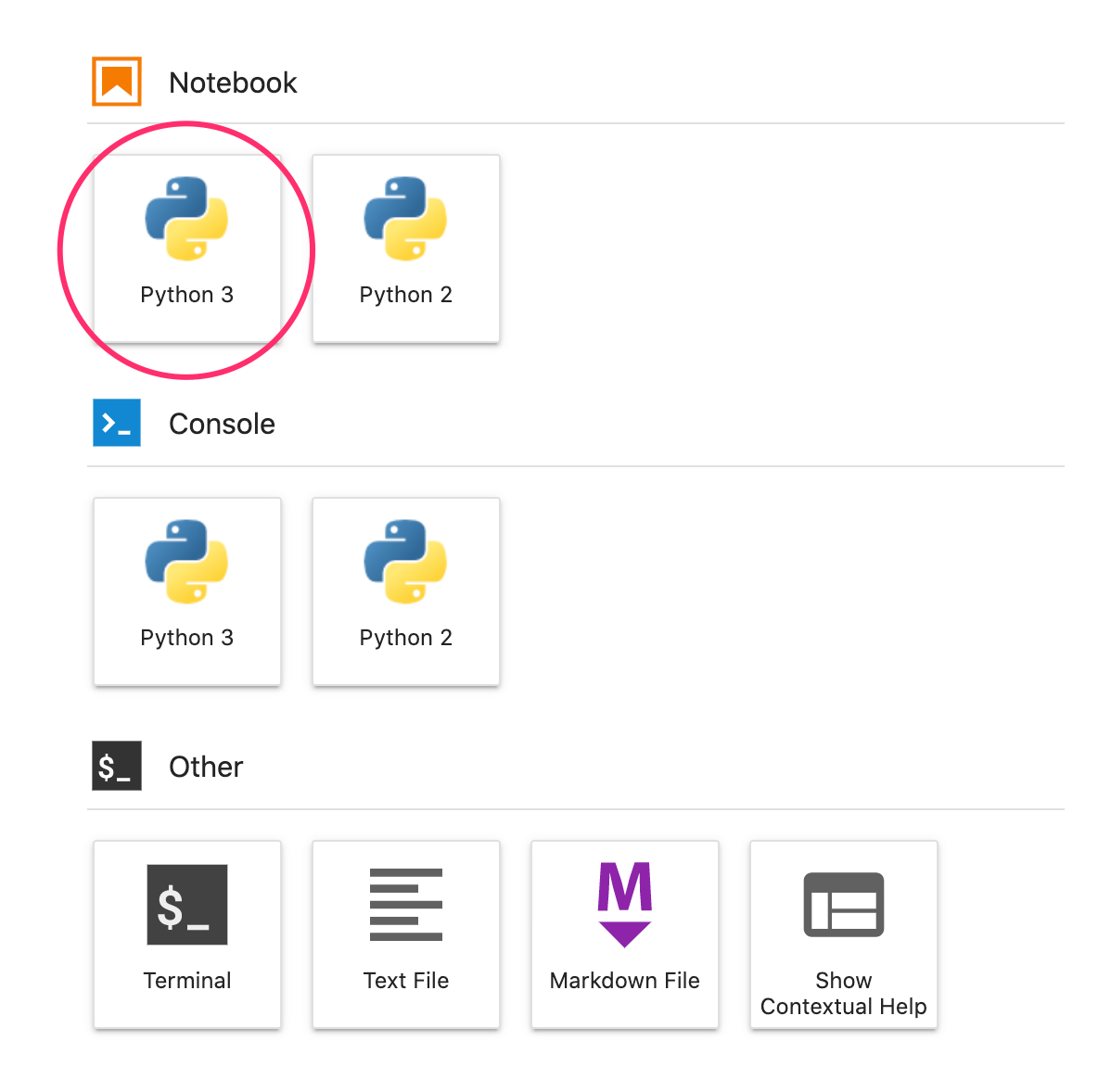You're ready to get started!

## Step 5: Import Python packages

In the first cell of your notebook, add the following imports and run the cell. You can run it by pressing the right arrow button in the top menu or pressing command-enter:

``````import datetime
import numpy as np
import os
import pandas as pd
import time
``````

You'll notice that we're not importing PyTorch here. This is because we're running the training job on AI Platform Training, not from our Notebook instance.

## 3. Create a package for the training job

To run our training job on AI Platform Training, we'll need our training code packaged locally in our Notebooks instance, and a Cloud Storage bucket to store assets for our job. First, we'll create a storage bucket. You can skip this step if you already have one.

## Step 1: Create a Cloud Storage bucket for our model

Let's first define some environment variables that we'll be using throughout the rest of the codelab. Fill in the values below with the name of your Google Cloud project and the name of the cloud storage bucket you'd like to create (must be globally unique):

``````# Update these to your own GCP project, model, and version names
GCP_PROJECT = 'your-gcp-project'
BOCKET_URL = 'gs://storage_bucket_name'
``````

Now we're ready to create a storage bucket, which we'll point to when we kick off our training job.

Run this `gsutil` command from within your notebook to create a bucket:

``````!gsutil mb \$BUCKET_URL
``````

## Step 2: Create the initial files for our Python package

To run a training job on AI Platform, we'll need to configure our code as a Python package. This consists of a `setup.py` file in our root directory that specifies any external package dependencies, a subdirectory with the name of our package (here we'll call it `trainer/`), and an empty `__init__.py` file within this subdirectory.

First, let's write our setup.py file. We're using the iPython %%writefile magics to save the file to our instance. Here we've specified 3 external libraries we'll be using in our training code: PyTorch, Scikit-learn, and Pandas:

``````%%writefile setup.py
from setuptools import find_packages
from setuptools import setup

REQUIRED_PACKAGES = ['torch>=1.5', 'scikit-learn>=0.20', 'pandas>=1.0']

setup(
name='trainer',
version='0.1',
install_requires=REQUIRED_PACKAGES,
packages=find_packages(),
include_package_data=True,
description='My training application package.'
)
``````

Next let's create our trainer/ directory and the empty init.py file within it. Python uses this file to recognize that this is a package:

``````!mkdir trainer
!touch trainer/__init__.py
``````

Now we're ready to start creating our training job.

## 4. Preview the dataset

The focus of this lab is on the tooling for training models here, but let's take a quick look at the dataset we'll be using to train our model to understand. We'll be using the natality dataset available in BigQuery. This contains birth data from the US over several decades. We'll be using a few columns from the dataset to predict a baby's birth weight. The original dataset is quite large, and we'll be using a subset of it that we've made available for you in a Cloud storage bucket.

Let's download the version of the dataset we've made available for you in Cloud Storage to a Pandas DataFrame and preview it.

``````natality = pd.read_csv('https://storage.googleapis.com/ml-design-patterns/natality.csv')
``````

This dataset has just under 100,000 rows. We'll be using 5 features to predict a baby's birth weight: mother and father age, gestation weeks, the mother's weight gain in pounds, and the baby's gender represented as a boolean.

## 5. Define the training job with hyperparameter tuning

We'll write our training script to a file called model.py within the trainer/ subdirectory we created earlier. Our training job will run on AI Platform Training, and it'll also make use of AI Platform's hyperparameter tuning service to find the optimal hyperparameters for our model utilizing Bayesian optimization.

## Step 1: Create the training script

First, let's create the Python file with our training script. Then we'll dissect what's happening in it. Running this %%writefile command will write the model code to a local Python file:

``````%%writefile trainer/model.py
import argparse
import hypertune
import numpy as np
import pandas as pd
import torch
import torch.nn as nn
import torch.optim as optim

from sklearn.utils import shuffle
from sklearn.preprocessing import LabelEncoder
from sklearn.preprocessing import normalize

def get_args():
"""Argument parser.
Returns:
Dictionary of arguments.
"""
parser = argparse.ArgumentParser(description='PyTorch MNIST')
parser.add_argument('--job-dir',  # handled automatically by AI Platform
help='GCS location to write checkpoints and export ' \
'models')
parser.add_argument('--lr',  # Specified in the config file
type=float,
default=0.01,
help='learning rate (default: 0.01)')
parser.add_argument('--momentum',  # Specified in the config file
type=float,
default=0.5,
help='SGD momentum (default: 0.5)')
parser.add_argument('--hidden-layer-size',  # Specified in the config file
type=int,
default=8,
help='hidden layer size')
args = parser.parse_args()
return args

def train_model(args):
# Get the data
natality = natality.dropna()
natality = shuffle(natality, random_state = 2)

natality_labels = natality['weight_pounds']
natality = natality.drop(columns=['weight_pounds'])

train_size = int(len(natality) * 0.8)
traindata_natality = natality[:train_size]
trainlabels_natality = natality_labels[:train_size]

testdata_natality = natality[train_size:]
testlabels_natality = natality_labels[train_size:]

# Normalize and convert to PT tensors
normalized_train = normalize(np.array(traindata_natality.values), axis=0)
normalized_test = normalize(np.array(testdata_natality.values), axis=0)

train_x = torch.Tensor(normalized_train)
train_y = torch.Tensor(np.array(trainlabels_natality))

test_x = torch.Tensor(normalized_test)
test_y = torch.Tensor(np.array(testlabels_natality))

# Define our data loaders
train_dataset = torch.utils.data.TensorDataset(train_x, train_y)

test_dataset = torch.utils.data.TensorDataset(test_x, test_y)

# Define the model, while tuning the size of our hidden layer
model = nn.Sequential(nn.Linear(len(train_x), args.hidden_layer_size),
nn.ReLU(),
nn.Linear(args.hidden_layer_size, 1))
criterion = nn.MSELoss()

# Tune hyperparameters in our optimizer
optimizer = optim.SGD(model.parameters(), lr=args.lr, momentum=args.momentum)
epochs = 20
for e in range(epochs):
for batch_id, (data, label) in enumerate(train_dataloader):
y_pred = model(data)
label = label.view(-1,1)
loss = criterion(y_pred, label)

loss.backward()
optimizer.step()

val_mse = 0
num_batches = 0
# Evaluate accuracy on our test set
for i, (data, label) in enumerate(test_dataloader):
num_batches += 1
y_pred = model(data)
mse = criterion(y_pred, label.view(-1,1))
val_mse += mse.item()

avg_val_mse = (val_mse / num_batches)

# Report the metric we're optimizing for to AI Platform's HyperTune service
# In this example, we're mimizing error on our test set
hpt = hypertune.HyperTune()
hpt.report_hyperparameter_tuning_metric(
hyperparameter_metric_tag='val_mse',
metric_value=avg_val_mse,
global_step=epochs
)

def main():
args = get_args()
print('in main', args)
train_model(args)

if __name__ == '__main__':
main()
``````

The training job consists of two functions where the bulk of the work is happening.

• `get_args()`: This parses the command line arguments we'll pass when we create our training job, along with the hyperparameters we want AI Platform to optimize. In this example our list of arguments includes only the hyperparameters we'll be optimizing – our model's learning rate, momentum, and the number of neurons in our hidden layer.
• `train_model()`: Here we download the data to a Pandas DataFrame, normalize it, convert it to PyTorch Tensors, and then define our model. To build our model we're using the PyTorch `nn.Sequential` API, which lets us define our model as a stack of layers:
``````model = nn.Sequential(nn.Linear(len(train_x), args.hidden_layer_size),
nn.ReLU(),
nn.Linear(args.hidden_layer_size, 1))
``````

Notice that instead of hardcoding the size of our model's hidden layer, we're making this a hyperparameter that AI Platform will tune for us. More on that in the next section.

## Step 2: Using AI Platform's hyperparameter tuning service

Instead of manually trying different hyperparameter values and retraining our model each time, we'll use Cloud AI Platform's hyperparameter optimization service. If we set up our training job with hyperparameter arguments, AI Platform will use Bayesian optimization to find the ideal values for the hyperparameters we specify.

In hyperparameter tuning, a single trial consists of one training run of our model with a specific combination of hyperparameter values. Depending on how many trials we run, AI Platform will use the results of completed trials to optimize the hyperparameters it selects for future ones. In order to configure hyperparameter tuning, we need to pass a config file when we kick off our training job with some data on each of the hyperparameters we're optimizing.

Next, create that config file locally:

``````%%writefile config.yaml
trainingInput:
hyperparameters:
goal: MINIMIZE
maxTrials: 10
maxParallelTrials: 5
hyperparameterMetricTag: val_mse
enableTrialEarlyStopping: TRUE
params:
- parameterName: lr
type: DOUBLE
minValue: 0.0001
maxValue: 0.1
scaleType: UNIT_LINEAR_SCALE
- parameterName: momentum
type: DOUBLE
minValue: 0.0
maxValue: 1.0
scaleType: UNIT_LINEAR_SCALE
- parameterName: hidden-layer-size
type: INTEGER
minValue: 8
maxValue: 32
scaleType: UNIT_LINEAR_SCALE
``````

For each hyperparameter, we specify the type, the range of values we'd like to search, and the scale on which to increase the value across different trials.

At the beginning of the job we also specify the metric we're optimizing for. Notice that at the end of our `train_model()` function above, we report this metric to AI Platform each time a trial completes. Here we're minimizing our model's mean squared error, and so we want to use the hyperparameters that result in the lowest mean squared error for our model. The name of this metric (`val_mse`) matches the name we use to report it when we call `report_hyperparameter_tuning_metric()` at the end of a trial.

## 6. Run a training job on AI Platform

In this section we'll kick off our model training job with hyperparameter tuning on AI Platform.

## Step 1: Define some environment variables

Let's first define some environment variables that we'll use to kick off our training job. If you'd like to run your job in a different region, update the REGION variable below:

``````MAIN_TRAINER_MODULE = "trainer.model"
TRAIN_DIR = os.getcwd() + '/trainer'
JOB_DIR = BUCKET_URL + '/output'
REGION = "us-central1"
``````

Each training job on AI Platform should have a unique name. Run the following to define a variable for the name of your job using a timestamp:

``````timestamp = str(datetime.datetime.now().time())
JOB_NAME = 'caip_training_' + str(int(time.time()))
``````

## Step 2: Kick off the training job

We'll create our training job using gcloud, the Google Cloud CLI. We can run this command directly in our notebook, referencing the variables we defined above:

``````!gcloud ai-platform jobs submit training \$JOB_NAME \
--scale-tier basic \
--package-path \$TRAIN_DIR \
--module-name \$MAIN_TRAINER_MODULE \
--job-dir \$JOB_DIR \
--region \$REGION \
--runtime-version 2.1 \
--python-version 3.7 \
--config config.yaml
``````

If your job was created correctly, head over to the Jobs section of your AI Platform console to monitor the logs.

## Step 3: Monitor your job

Once you're in the Jobs section of the console, click on the job you just started to view details: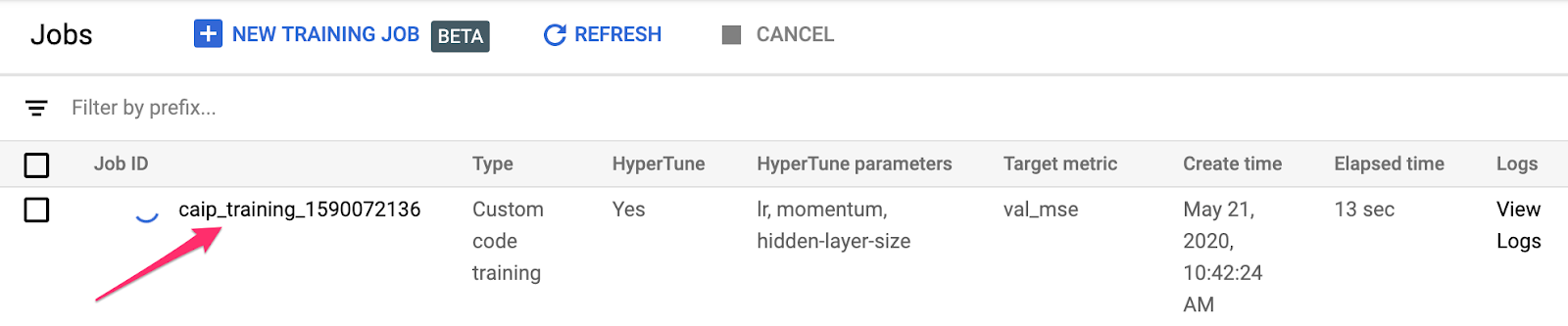As your first round of trials kicks off, you'll be able to see the hyperparameter values selected for each trial: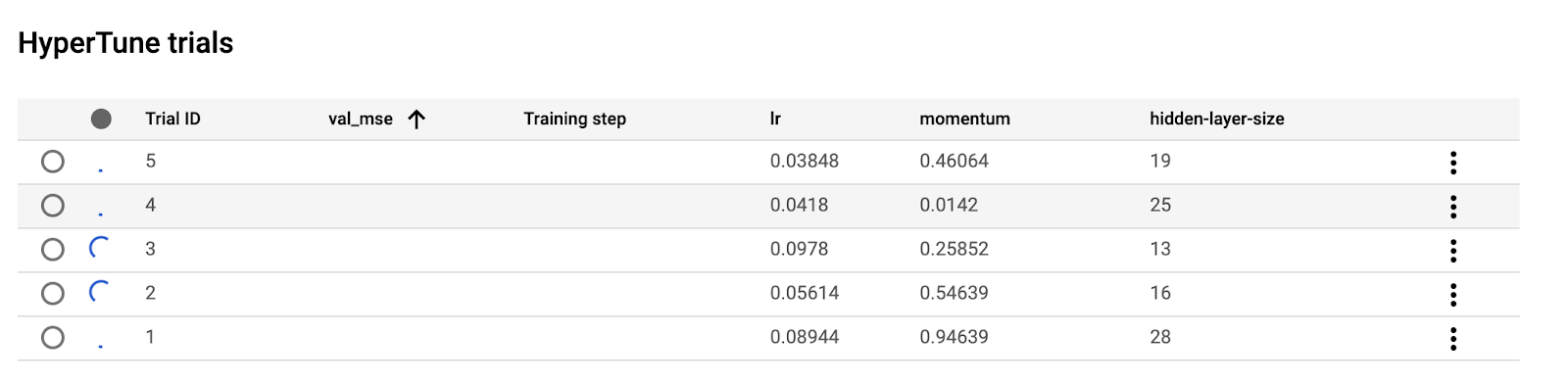As trials complete, the resulting value of your optimization metric (in this case `val_mse`) will be logged here. The job should take 15-20 minutes to run, and the dashboard will look something like this when the job has finished (exact values will vary):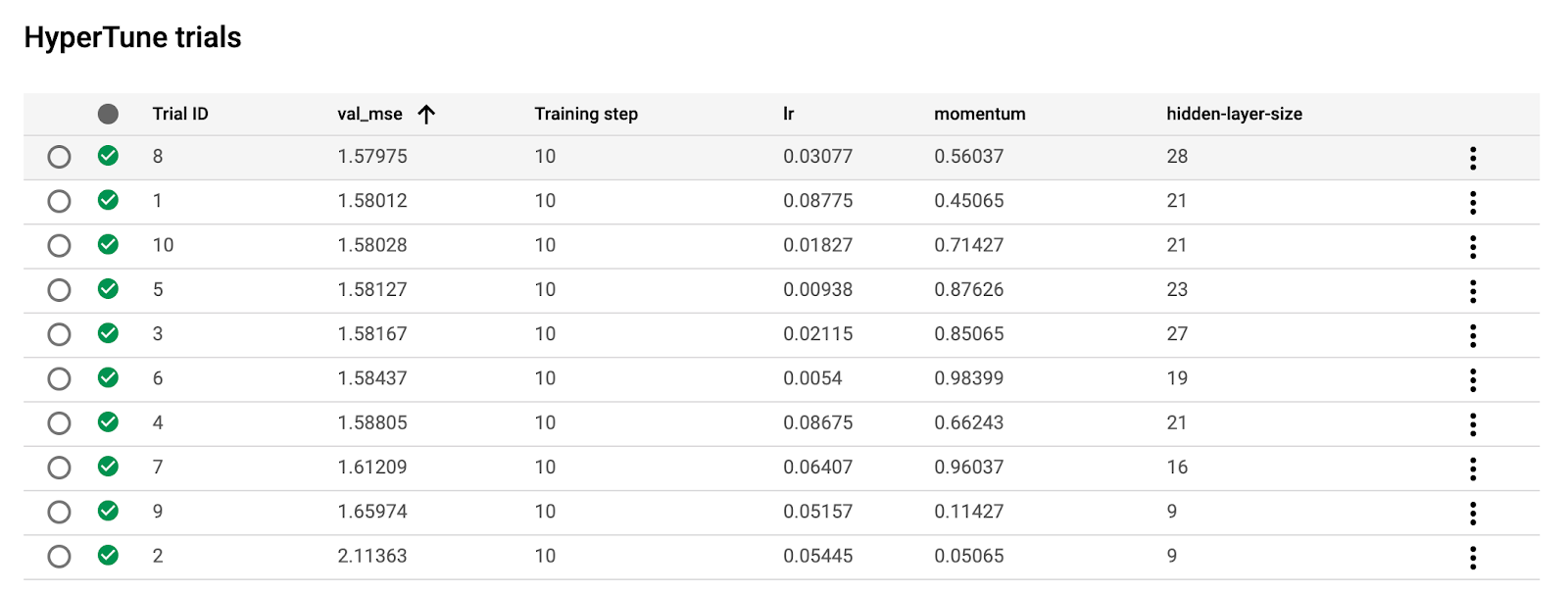To debug potential issues and monitor your job in more detail, click on View Logs from the jobs detail page: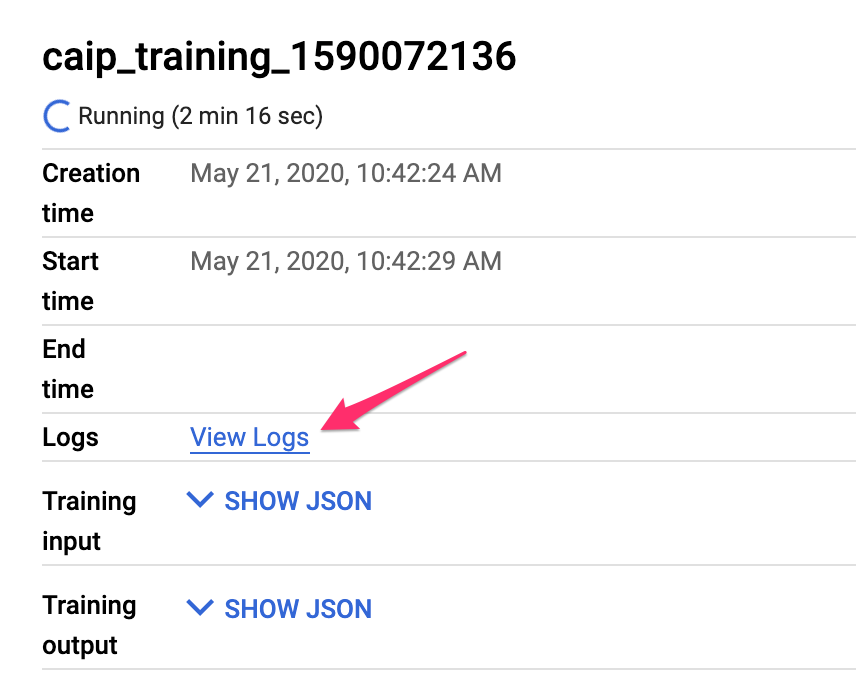Every `print()` statement in your model training code will show up here. If you're running into issues, try adding more print statements and starting a new training job.

Once your training job completes, find the hyperparameters that yielded the lowest val_mse. You can either use these to train and export a final version of your model, or use them as guidance to kick off another training job with additional hyperparameter tuning trials.

## 7. Cleanup

If you'd like to continue using this notebook, it is recommended that you turn it off when not in use. From the Notebooks UI in your Cloud Console, select the notebook and then select Stop: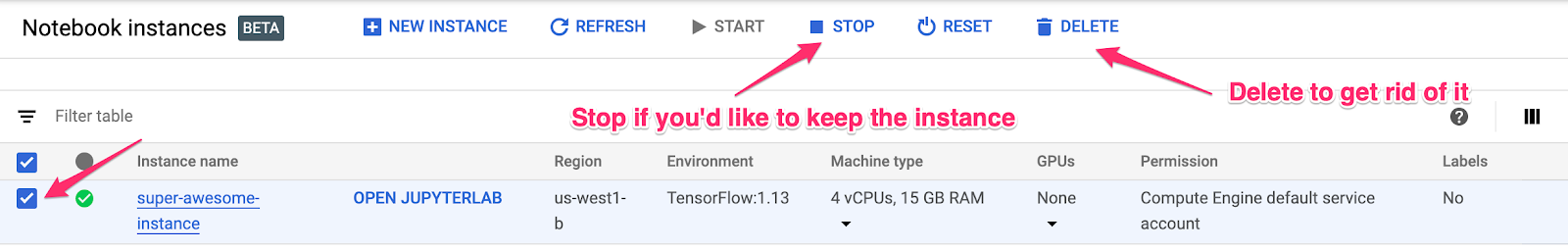If you'd like to delete all resources you've created in this lab, simply delete the notebook instance instead of stopping it.

Using the Navigation menu in your Cloud Console, browse to Storage and delete both buckets you created to store your model assets.

[{ "type": "thumb-down", "id": "missingTheInformationINeed", "label":"Missing the information I need" },{ "type": "thumb-down", "id": "tooComplicatedTooManySteps", "label":"Too complicated / too many steps" },{ "type": "thumb-down", "id": "outOfDate", "label":"Out of date" },{ "type": "thumb-down", "id": "samplesCodeIssue", "label":"Samples / code issue" },{ "type": "thumb-down", "id": "otherDown", "label":"Other" }]
[{ "type": "thumb-up", "id": "easyToUnderstand", "label":"Easy to understand" },{ "type": "thumb-up", "id": "solvedMyProblem", "label":"Solved my problem" },{ "type": "thumb-up", "id": "otherUp", "label":"Other" }]